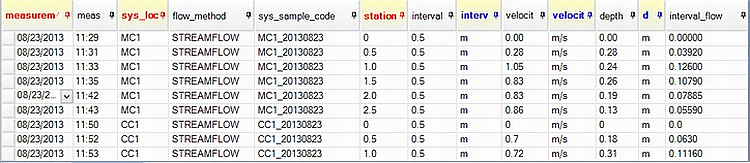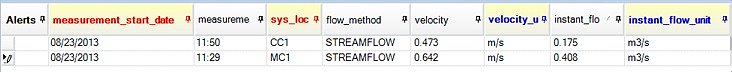﻿ Streamflow Method

# Streamflow Method

Navigation:  EDGE > EDGE Features > Flow Calculations >

# Streamflow Method

This method calculates the instant flow (m3/sec) within a stream at a given location, by summing the flow measurements of a number of subsections of the stream at a specific location using actual field measurements of subsection width, subsection depth and subsection flow velocity.With this method, subsection measurements are made at each location (SYS_LOC_CODE) for depth (m), width (m) and velocity (m/sec) and input into the FLOW_MEASUREMENT tab in EDGE or the EDD. The ‘interval flow’ or ‘discharge’ for each subsection is calculated by EDP or EDGE, using the equations:

Equation B. i)  Subsection Discharge, m3/sec (interval_flow) = (Subsection Depth (m) X Subsection Width  (m)) X Subsection Velocity (m/sec)

The ‘instant flow’ or discharge for the location (SYS_LOC_CODE) is calculated using the equation:

Equation B. ii)  Instant Flow (m3/sec) = Sum (interval_flow) for a specific date and SYS_LOC_CODE combination.

1.Populate EDD in EDGE or in Excel.

i.in EDGE: open the ‘Flow_Measurement’ tab.

i.Enter field measurements for a specific location (SYS_LOC_CODE) and date.

ii.Select 'STREAMFLOW' as the FLOW_METHOD.

iii.Measurements and units for STATION, INTERVAL, VELOCITY and DEPTH must be entered.

iv.The column INTERVAL_FLOW will automatically populate on entry of these fields.

v.Note that The MEASUREMENT_DATE_TIME and LOCATION_CODE can be identical as long as the STATION is unique.  A row will automatically be created in the ‘Flow’ tab for each unique date and SYS_LOC_CODE combination.

vi.A value for FLOW.INSTANT_FLOW will automatically be populated based on the equation ii) above.

vii.A value for FLOW.VELOCITY will be automatically populated based on the equation:

Equation B. iii) FLOW.VELOCITY = Average FLOW_MEASUREMENT.VELOCITY for each unique SYS_LOC_CODE and date combination.Example of EDGE Flow Interface

ii.In Excel:

i.the FLOW_MEASUREMENT values should be entered in the ‘Flow_Measurement’ tab of the flow EDD.

ii.Measurements and units for STATION, INTERVAL, VELOCITY and DEPTH must be entered.

iii.A value for FLOW_MEASUREMENT.INTERVAL_FLOW must also be calculated for the FLOW.INSTANT_FLOW column to be populated (see Equation B.i) above).

2.Open EDD in EDP. When the EDD is opened, EDP will automatically create and populate certain fields in the Flow tab (if not already calculated by EDGE):

i.A row will automatically be created in Flow for each unique date and SYS_LOC_CODE combination.

ii.A value for ‘FLOW.INSTANT_FLOW’ will automatically populate based on the equation B.ii) above.

iii.A value for FLOW.VELOCITY will automatically populate based on the equation B. iii) above.

iv.The FLOW.REMARK column will be populated with ‘EDP populated instant_flow; EDP populated velocity’ to confirm this.

3.The data can then be created and committed, which will populate the EQuIS tables DT_FLOW, DT_FLOW_MEASUREMENT, DT_SAMPLE and DT_FIELD_SAMPLE.